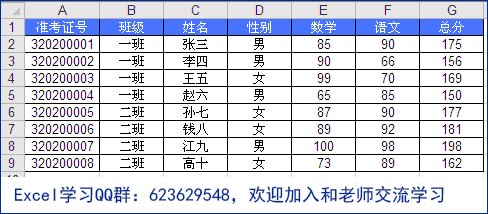# excel条件函数各版本实例汇总

[日期：2011-09-10]   来源：IT部落窝  作者：IT部落窝   阅读：4011[字体： ]

Excel条件函数在实际中使用比较常见，对数据按照条件进行运算汇总。

Excel条件函数大概有：IF逻辑条件函数，COUNTIF条件计数，SUMIF条件求和，SUMPRODUCT多条件计数求和。

在Excel 2007以后的版本，又增加了AVERAGEIF、AVERAGEIFS、SUMIFS、COUNTIFS和IFERROR五个函数，它们都可以在一定范围内根据条件计数和求和。特别是多重条件函数AVERAGEIFS、COUNTIFS和 SUMIFS给工作带来了极大的方便。

上面提到的这些Excel条件函数，为运算添加了“条件”，下面通过几个实例介绍之。通过上表中的数据源，完成下面4题。

第一题：求一班的数学平均分
用到的excel条件函数有：COUNTIF，SUMIF，AVERAGEIF。提供三种解法：
数组公式：=SUMIF(B2:B9,"一班",E2:E9)/COUNTIF(B2:B9,"一班")
数组公式：=AVERAGE(IF(B2:B9="一班",E2:E9))
=AVERAGEIF(B2:B9,"一班",E2:E9)

第二题：求一班男生的数学平均分
用到的excel条件函数有：IF，SUMPRODUCT，AVERAGEIFS。提供三种解法：
数组公式：=AVERAGE(IF(B2:B9="一班",IF(D2:D9="男",E2:E9)))
=SUMPRODUCT((B2:B9="一班")*(D2:D9="男")*F2:F9)/SUMPRODUCT((B2:B9="一班")*(D2:D9="男"))
=AVERAGEIFS(F2:F9,D2:D9,"男",B2:B9,"一班")

第三题：求一班男生的语文总分
用到的excel条件函数有：SUMIFS，SUM。提供两种解法：
数组公式：=SUM((B2:B9="一班")*(D2:D9="男")*F2:F9)
=SUMIFS(F2:F9,B2:B9,"一班",D2:D9,"男")

第四题：求一班男生的人数
用到的excel条件函数有：COUNTIFS，SUM。提供两种解法：
数组公式：=SUM((B2:B9="一班")*(D2:D9="男"))
=COUNTIFS(B2:B9,"一班",D2:D9,"男")

上面这些条件求和、计数、平均，除了使用Excel的条件函数以外，也可以使用基本功能来实现。主要有：筛选、分类汇总、数据透视表、多条件求和向导。分享到： QQ空间 新浪微博 腾讯微博 人人网